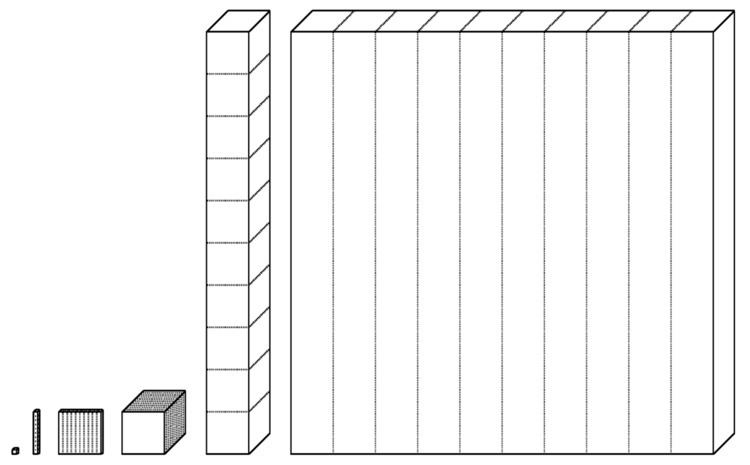# Savart

Updated on
Covid-19The savart /səˈvɑːr/ is a unit of measurement for musical pitch intervals ( play ). One savart is equal to one thousandth of a decade (10/1: 3,986.313714 cents): 3.9863 cents. Musically, in just intonation, the interval of a decade is precisely a just major twenty-fourth, or, in other words, three octaves and a just major third. Today the savart has largely been replaced by the cent and the millioctave. The savart is practically the same as the earlier heptameride (eptameride), one seventh of a meride. One tenth of an heptameride is a decameride and a hundredth of an heptameride (thousandth of a decade) is a jot.

## Definition

If f 2 f 1 is the ratio of frequencies of a given interval, the corresponding measure in savarts is given by:

s = 1000 log 10 f 2 f 1

or

f 2 f 1 = 10 s / 1000

Like the more common cent, the savart is a logarithmic measure, and thus intervals can be added by simply adding their savart values, instead of multiplying them as you would frequencies. The number of savarts in an octave is 1000 times the base-10 logarithm of 2, or nearly 301.03. Sometimes this is rounded to 300, which makes the unit more useful for equal temperament.

## Conversion

The conversion from savarts into cents or millioctaves is:

1   s a v a r t = 1.2 log 10 2   c e n t 3.9863   c e n t

1   s a v a r t = 1 log 10 2   m i l l i o c t a v e 3.3219   m i l l i o c t a v e

## History

The savart is named after the French physicist and doctor Félix Savart (1791–1841) who advocated the earlier similar interval of the French acoustician Joseph Sauveur (1653–1716). Sauveur proposed the méride, eptaméride (or heptaméride), and decaméride. In English these are meride, heptameride, and decameride respectively. The octave is divided into 43 merides, the meride is divided into seven heptamerides, and the heptameride is divided into ten decamerides. There are thus 43 × 7 = 301 heptamerides in an octave. The attraction of this scheme to Sauveur was that log10(2) is very close to .301, and thus the number of heptamerides in a given ratio is found to a high degree of accuracy from simply its log times 1000. This is equivalent to assuming 1000 heptamerides in a decade rather than 301 in an octave, the same as Savart's definition. The unit was given the name savart sometime in the 20th century. A disadvantage of this scheme is that there are not an exact number of heptamerides/savarts in an equal tempered semitone. For this reason Alexander Wood used a modified definition of the savart, with 300 savarts in an octave, and hence 25 savarts in a semitone.

A related unit is the jot, of which there are 30103 in an octave, or approximately 100,000 in a decade. The jot is defined in a similar way to the savart, but has a more accurate rounding of log10(2) because more digits are used. There are approximately 100 jots in a savart. The unit was first described by Augustus de Morgan (1806-1871) which he called an atom. The name jot was coined by John Curwen (1816-1880) at the suggestion of Hermann von Helmholtz.

Topics